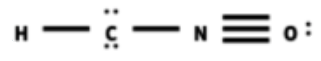# Problem: Draw a Lewis structure and add formal charges to each resonance form of HCNO.

###### FREE Expert Solution
• N : prefers a complete octet, more likely to have a +1 formal charge (more stable)
• O : prefers a complete octet, more likely to have a -1 formal charge (more stable)
• C : prefers a complete octet, more likely to have a 0 formal charge (more stable)

a)Formal charge of N

F.C. of N = 5 – (4 + 0) = +1

Formal charge of O

F.C. of O = 6 – (3 + 2) = +1

Formal charge of C

F.C. of C = 4 – (2 + 4) = -2

Overall charge

Overall = 1+1-2 = 0

86% (175 ratings)###### Problem Details

Draw a Lewis structure and add formal charges to each resonance form of HCNO.

Frequently Asked Questions

What scientific concept do you need to know in order to solve this problem?

Our tutors have indicated that to solve this problem you will need to apply the Resonance Structures concept. You can view video lessons to learn Resonance Structures. Or if you need more Resonance Structures practice, you can also practice Resonance Structures practice problems.

What professor is this problem relevant for?

Based on our data, we think this problem is relevant for Professor McWhorter's class at CLEMSON.